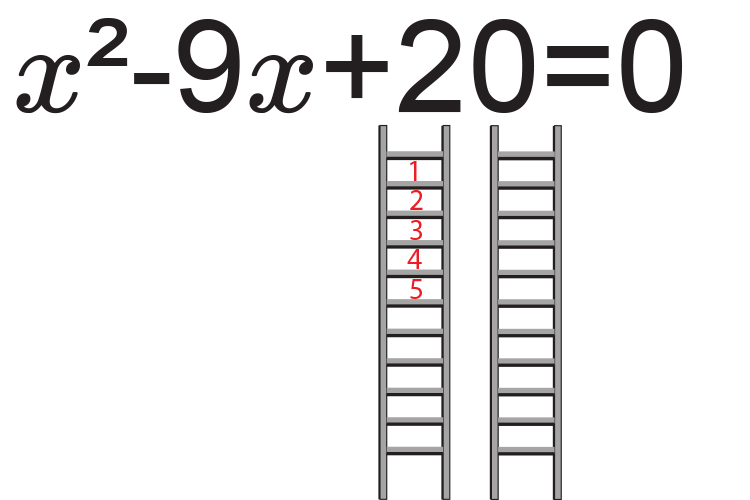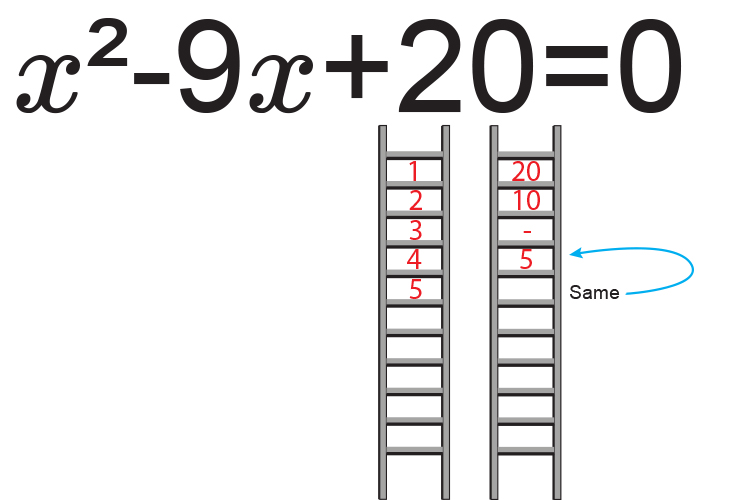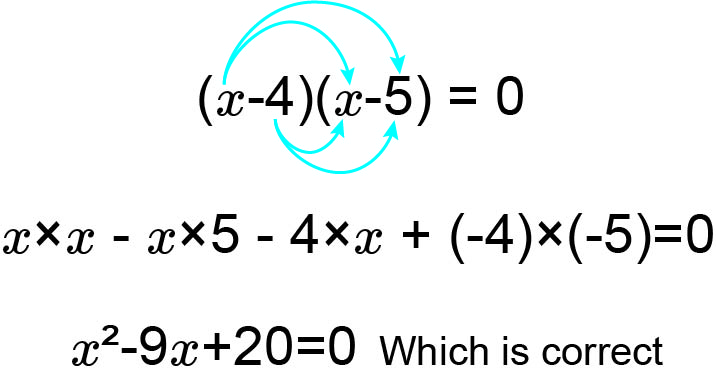# Factorising quadratics - (easy) Example 2

Factorise the quadratic x^2-9x+20=0The factors of 20 are:

1 and 20

2 and 10

4 and 5

Now ask yourself what factors of c  add to give b.

i.e. what factors of 20  equal -9.

-4times-5=20           -4-5=-9

Therefore:

x^2-9x+20=0

Can be written as:

(x-4)(x-5)

Check by multiplying out:The roots of x^2-9x+20=0

are (x-4)=0

and (x-5)=0

Now solve for x

x=4   or  x=5

The roots of x^2-9x+20=0  are x=4  or x=5